Home > CC2 > Chapter 9 > Lesson 9.2.1 > Problem9-60

9-60.
1. Find the area and perimeter of each shape below. Show your steps and work. Homework Help ✎

1. All angles are right angles.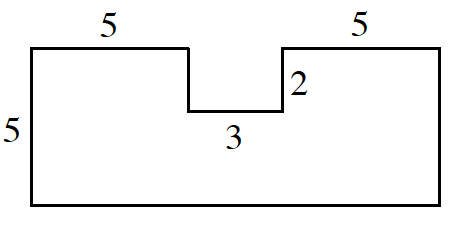2.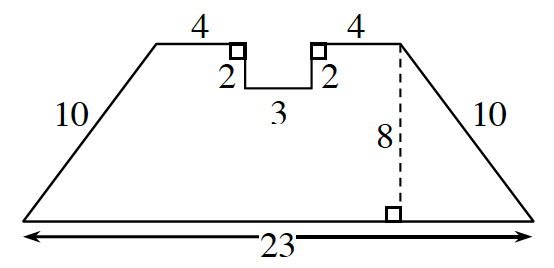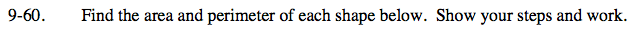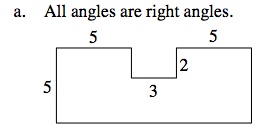Label the missing sides.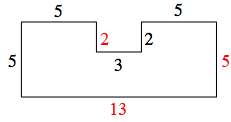One method for calculating the area is to think of the shape as a large rectangle with a small (2 × 3) rectangle missing.

Perimeter = 40 units
Area = 65 − 6 = 59 units2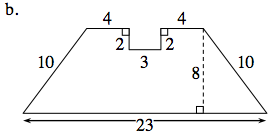Area of a trapezoid.

$\text{Area}=\left(\frac{1}{2}\right)(\text{base}_1+\text{base}_2)(\text{height})$